Fluid Dynamics and Its Biological and Medical Applications

# Motion of an Object in a Viscous Fluid

OpenStaxCollege

### Learning Objectives

• Calculate the Reynolds number for an object moving through a fluid.
• Explain whether the Reynolds number indicates laminar or turbulent flow.
• Describe the conditions under which an object has a terminal speed.

A moving object in a viscous fluid is equivalent to a stationary object in a flowing fluid stream. (For example, when you ride a bicycle at 10 m/s in still air, you feel the air in your face exactly as if you were stationary in a 10-m/s wind.) Flow of the stationary fluid around a moving object may be laminar, turbulent, or a combination of the two. Just as with flow in tubes, it is possible to predict when a moving object creates turbulence. We use another form of the Reynolds number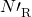, defined for an object moving in a fluid to be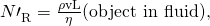where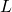is a characteristic length of the object (a sphere’s diameter, for example),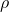the fluid density,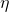its viscosity, and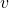the object’s speed in the fluid. Ifis less than about 1, flow around the object can be laminar, particularly if the object has a smooth shape. The transition to turbulent flow occurs forbetween 1 and about 10, depending on surface roughness and so on. Depending on the surface, there can be a turbulent wake behind the object with some laminar flow over its surface. For anbetween 10 and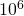, the flow may be either laminar or turbulent and may oscillate between the two. Forgreater than about, the flow is entirely turbulent, even at the surface of the object. (See [link].) Laminar flow occurs mostly when the objects in the fluid are small, such as raindrops, pollen, and blood cells in plasma.

Does a Ball Have a Turbulent Wake?

Calculate the Reynolds numberfor a ball with a 7.40-cm diameter thrown at 40.0 m/s.

Strategy

We can use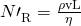to calculate, since all values in it are either given or can be found in tables of density and viscosity.

Solution

Substituting values into the equation foryields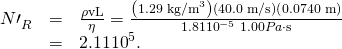Discussion

This value is sufficiently high to imply a turbulent wake. Most large objects, such as airplanes and sailboats, create significant turbulence as they move. As noted before, the Bernoulli principle gives only qualitatively-correct results in such situations.

One of the consequences of viscosity is a resistance force called viscous drag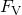that is exerted on a moving object. This force typically depends on the object’s speed (in contrast with simple friction). Experiments have shown that for laminar flow (less than about one) viscous drag is proportional to speed, whereas forbetween about 10 and, viscous drag is proportional to speed squared. (This relationship is a strong dependence and is pertinent to bicycle racing, where even a small headwind causes significantly increased drag on the racer. Cyclists take turns being the leader in the pack for this reason.) Forgreater than, drag increases dramatically and behaves with greater complexity. For laminar flow around a sphere,is proportional to fluid viscosity, the object’s characteristic size, and its speed. All of which makes sense—the more viscous the fluid and the larger the object, the more drag we expect. Recall Stoke’s law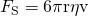. For the special case of a small sphere of radius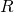moving slowly in a fluid of viscosity, the drag force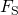is given by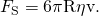(a) Motion of this sphere to the right is equivalent to fluid flow to the left. Here the flow is laminar withless than 1. There is a force, called viscous drag, to the left on the ball due to the fluid’s viscosity. (b) At a higher speed, the flow becomes partially turbulent, creating a wake starting where the flow lines separate from the surface. Pressure in the wake is less than in front of the sphere, because fluid speed is less, creating a net force to the left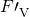that is significantly greater than for laminar flow. Hereis greater than 10. (c) At much higher speeds, whereis greater than, flow becomes turbulent everywhere on the surface and behind the sphere. Drag increases dramatically.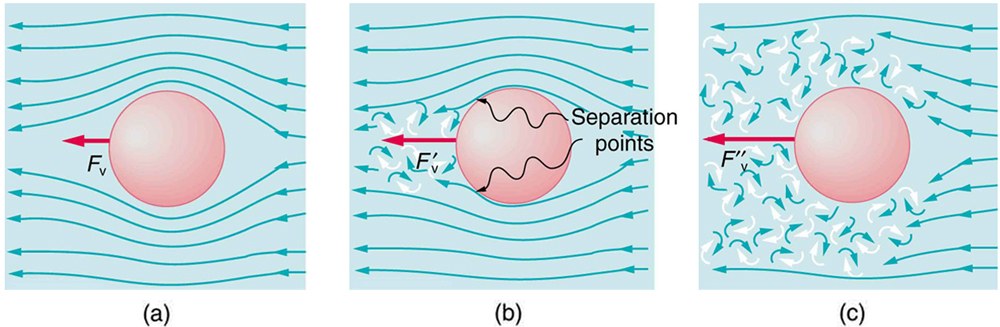An interesting consequence of the increase inwith speed is that an object falling through a fluid will not continue to accelerate indefinitely (as it would if we neglect air resistance, for example). Instead, viscous drag increases, slowing acceleration, until a critical speed, called the terminal speed, is reached and the acceleration of the object becomes zero. Once this happens, the object continues to fall at constant speed (the terminal speed). This is the case for particles of sand falling in the ocean, cells falling in a centrifuge, and sky divers falling through the air. [link] shows some of the factors that affect terminal speed. There is a viscous drag on the object that depends on the viscosity of the fluid and the size of the object. But there is also a buoyant force that depends on the density of the object relative to the fluid. Terminal speed will be greatest for low-viscosity fluids and objects with high densities and small sizes. Thus a skydiver falls more slowly with outspread limbs than when they are in a pike position—head first with hands at their side and legs together.

Take-Home Experiment: Don’t Lose Your Marbles

By measuring the terminal speed of a slowly moving sphere in a viscous fluid, one can find the viscosity of that fluid (at that temperature). It can be difficult to find small ball bearings around the house, but a small marble will do. Gather two or three fluids (syrup, motor oil, honey, olive oil, etc.) and a thick, tall clear glass or vase. Drop the marble into the center of the fluid and time its fall (after letting it drop a little to reach its terminal speed). Compare your values for the terminal speed and see if they are inversely proportional to the viscosities as listed in [link]. Does it make a difference if the marble is dropped near the side of the glass?

Knowledge of terminal speed is useful for estimating sedimentation rates of small particles. We know from watching mud settle out of dirty water that sedimentation is usually a slow process. Centrifuges are used to speed sedimentation by creating accelerated frames in which gravitational acceleration is replaced by centripetal acceleration, which can be much greater, increasing the terminal speed.

There are three forces acting on an object falling through a viscous fluid: its weight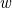, the viscous drag, and the buoyant force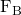.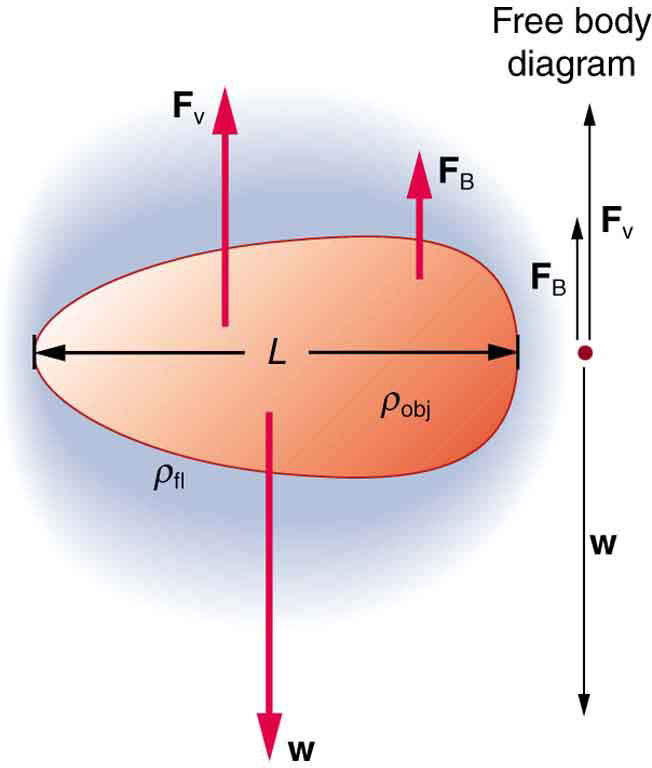# Section Summary

• When an object moves in a fluid, there is a different form of the Reynolds numberwhich indicates whether flow is laminar or turbulent.
• Forless than about one, flow is laminar.
• Forgreater than, flow is entirely turbulent.

# Conceptual Questions

What direction will a helium balloon move inside a car that is slowing down—toward the front or back? Explain your answer.

Will identical raindrops fall more rapidly in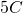air or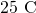air, neglecting any differences in air density? Explain your answer.

If you took two marbles of different sizes, what would you expect to observe about the relative magnitudes of their terminal velocities?

## Glossary

viscous drag
a resistance force exerted on a moving object, with a nontrivial dependence on velocity
terminal speed
the speed at which the viscous drag of an object falling in a viscous fluid is equal to the other forces acting on the object (such as gravity), so that the acceleration of the object is zero